常见的五种神经网络(3)-循环神经网络（上）篇

13 篇文章 7 订阅

Github：https://github.com/thinkgamer

引言

• 循环神经网络概述
• 如何给神经网络增加记忆能力
• 延时神经网络
• 有外部输入的非线性自回归模型
• 循环神经网络
• 一般的循环神经网络
• 单向循环神经网络
• 双向循环神经网络
• 深度循环神经网络
• 循环神经网络应用到机器学习任务

概述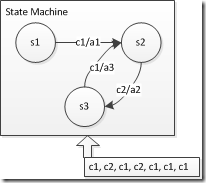如何给网络增加记忆能力

• 延时神经网络
• 有外部输入的非线性自回归模型
• 循环神经网络

延时神经网络

h t l + 1 = f ( h t l , h t − 1 l , . . . . , h t − p + 1 l ) h_t^{l+1} = f(h_t^l,h_{t-1}^l,....,h_{t-p+1}^l)

有外部输入的非线性自回归模型

y t = w 0 + ∑ i = 1 p w p ∗ y t − i + ε t y_t = w_0 + \sum_{i=1}^{p}w_p * y_{t-i} + \varepsilon_t

y t = f ( x t , x t − 1 , . . , x t − p , y t − 1 , y t − 2 , . . . . , y t − q ) y_t = f(x_t, x_{t-1},..,x_{t-p}, y_{t-1},y_{t-2},....,y_{t-q})

循环神经网络

h t = ( h t − 1 , x t ) h_t = (h_{t-1}, x_t)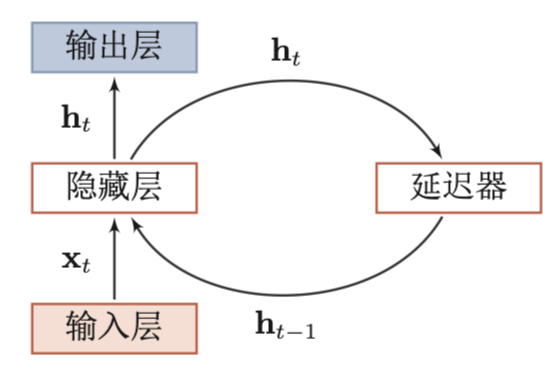一般的循环神经网络

• 单向循环神经网络
• 双向循环神经网络

• 循环神经网络
• 深度循环神经网络

单向循环神经网络

（图-2）所示即为一个单向的循环神经网络，对其展开后的效果图如下（图-3）：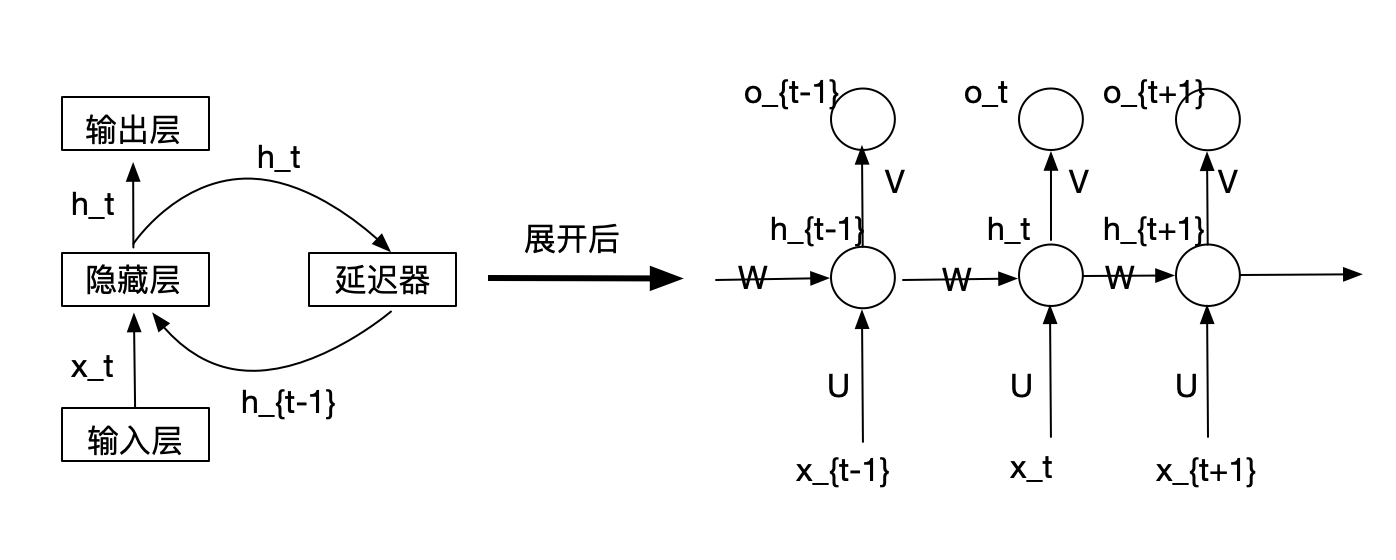o t = g ( V ∗ h t ) h t = f ( U ∗ x t + W ∗ h t − 1 ) o_t = g(V*h_t) \\ h_t = f(U*x_t + W*h_{t-1})

双向循环神经网络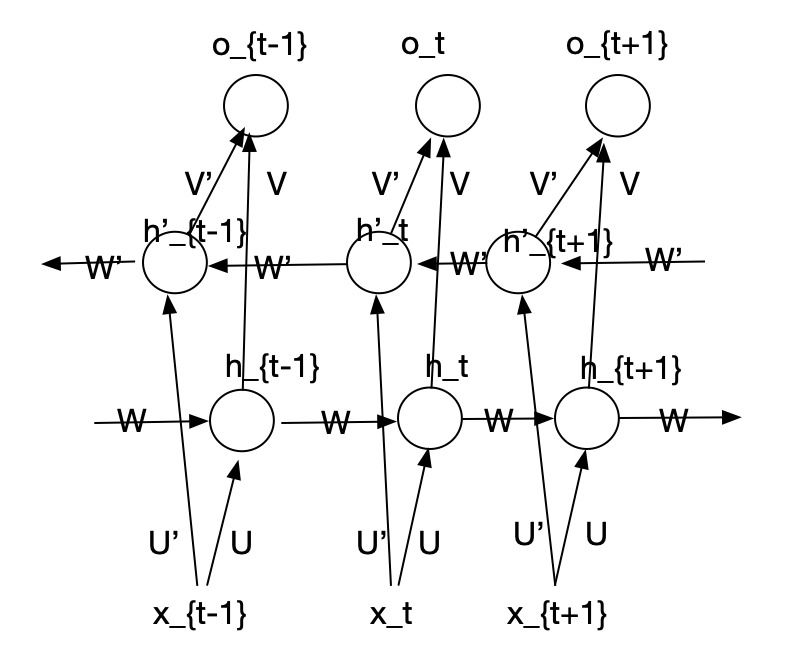o t = g ( V ∗ h t + V ′ ∗ h t ′ ) h t = f ( U ∗ x t + W ∗ h t − 1 ) h t ′ = f ( U ′ ∗ x t + W ′ ∗ h t + 1 ′ ) o_t = g(V*h_t + V'*h'_t) \\ h_t = f(U*x_t + W*h_{t-1}) \\ h'_t = f(U'*x_t + W'*h'_{t+1})

深度循环神经网络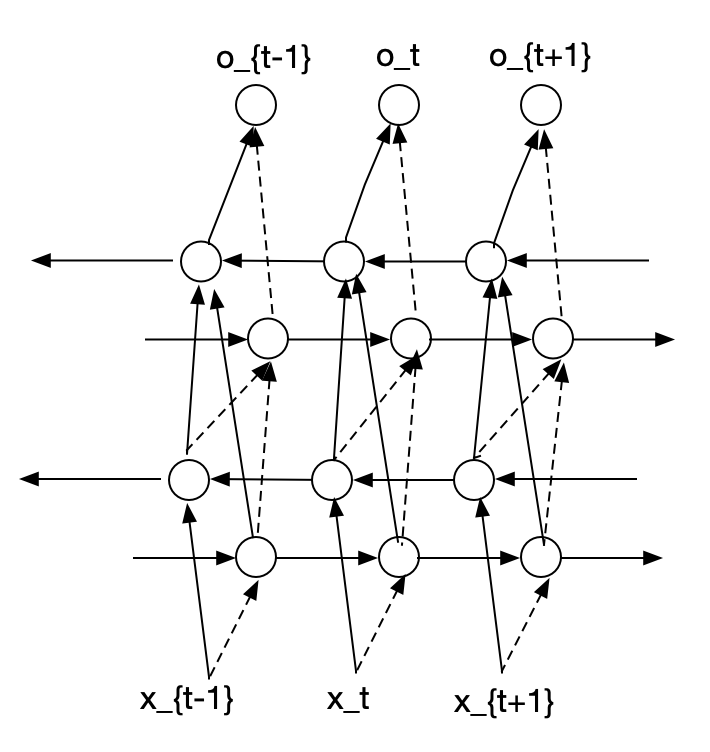o t = g ( V ( i ) ∗ h t ( i ) + V ′ ( i ) ∗ h t ′ ( i ) ) h t ( i ) = f ( U ( i ) ∗ h t ( i − 1 ) + W ( i ) ∗ h t − 1 ) h t ′ ( i ) = f ( U ′ ( i ) ∗ h t ′ ( i − 1 ) + W ′ ( i ) ∗ h t + 1 ′ ) . . . h t ( 1 ) = f ( U ( 1 ) ∗ h t + W ( 1 ) ∗ h t − 1 ) h t ′ ( 1 ) = f ( U ′ ( 1 ) ∗ h t + W ′ ( 1 ) ∗ h t + 1 ′ ) o_t = g(V^{(i)}*h^{(i)}_t + V'^{(i)}*h'^{(i)}_t) \\ h^{(i)}_t = f(U^{(i)}*h^{(i-1)}_t + W^{(i)}*h_{t-1}) \\ h'^{(i)}_t = f(U'^{(i)}*h'^{(i-1)}_t + W'^{(i)}*h'_{t+1}) \\ ... \\ h^{(1)}_t = f(U^{(1)} * h_t + W^{(1)}*h_{t-1}) \\ h'^{(1)}_t = f(U'^{(1)} * h_t + W'^{(1)}*h'_{t+1}) \\

循环神经网络应用到机器学习任务

• 序列到类别模式
• 同步的序列到序列模式
• 异步的序列到序列模式

序列到类别模式

y ^ = g ( h T ) \hat{y} = g(h_T)

y ^ = g ( 1 T ∑ t = 1 T h t ) \hat{y} = g( \frac{1}{T} \sum_{t=1}^{T} h_t )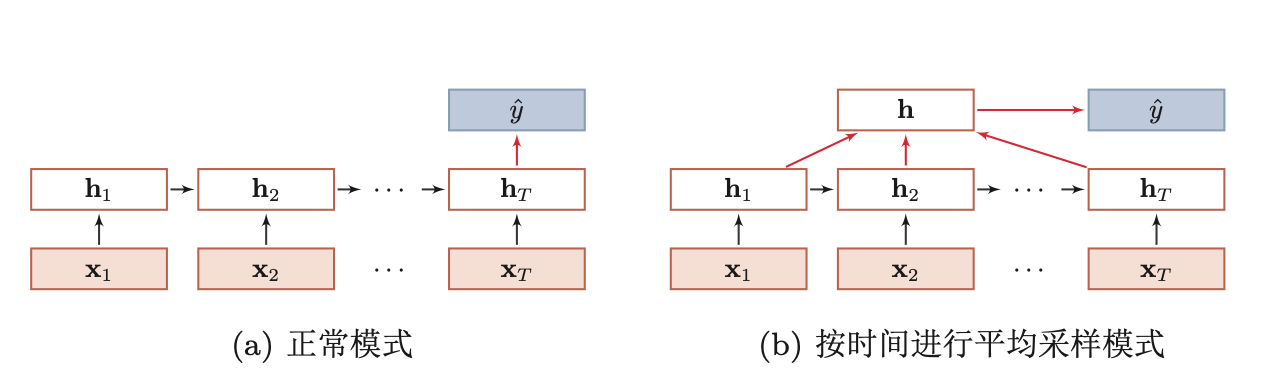同步的序列到序列模式

y ^ = g ( h T ) , ∀ t ∈ [ 1 , T ] \hat{y} = g(h_T), \forall_t \in [1,T]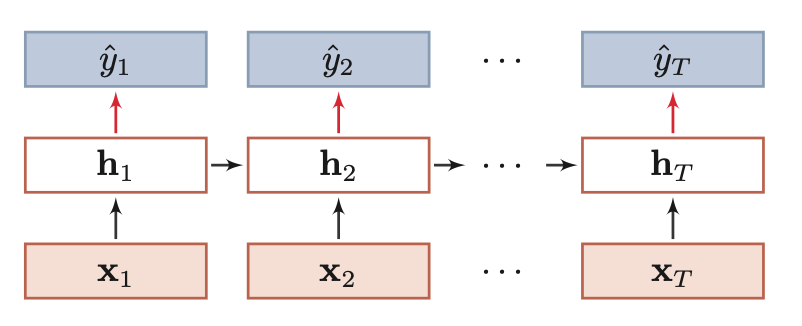异步的序列到序列模式

h t = f 1 ( h t − 1 , x t ) , ∀ t ∈ [ 1 , T ] h T + t = f 2 ( h T + t − 1 , x t ) , ∀ t ∈ [ 1 , M ] y ^ t = g ( h T + t ) , ∀ t ∈ [ 1 , M ] h_t = f_1(h_{t-1},x_t), \forall_t \in [1,T] \\ h_{T+t} = f_2(h_{T+t-1},x_t), \forall_t \in [1,M] \\ \hat{y} _t = g(h_{T+t}), \forall_t \in [1,M]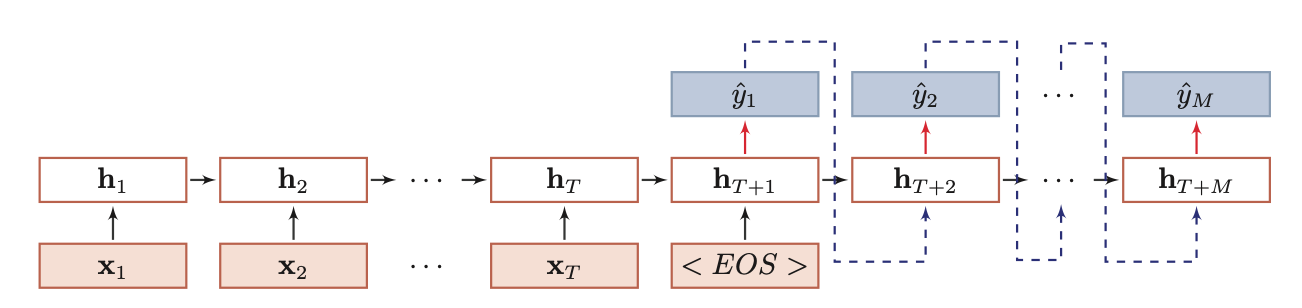【技术服务】，详情点击查看： https://mp.weixin.qq.com/s/PtX9ukKRBmazAWARprGIAg09-2301-182万+
08-03839
09-013万+
09-2347万+
01-31829
03-071万+
01-264873
09-113867
09-24701
05-2815万+
09-062万+
03-051084
09-182520
08-09453
10-31428
07-294033
08-076117¥2 ¥4 ¥6 ¥10 ¥20余额支付 (余额：-- )扫码支付获取中扫码支付点击重新获取扫码支付1.余额是钱包充值的虚拟货币，按照1:1的比例进行支付金额的抵扣。
2.余额无法直接购买下载，可以购买VIP、C币套餐、付费专栏及课程。余额充值